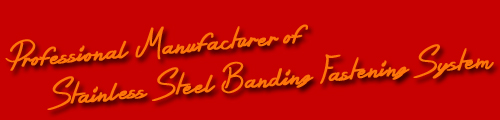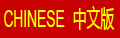Home > Chemical composition for AISI Standard stainless steel

# Chemical composition for AISI Standard stainless steel

MOWCO Industry Limited is a leading manufacturer in China of Stainless steel banding and wing seals, buckles.

 Type Chemical Composition Grade No. C Mn P S Si Cr Ni Mo Others AISI 201 <=0.15 5.50～7.50 <=0.06 <=0.03 <=0.75 16.0～18.0 3.5～5.5 - N<=0.25 AISI 202 <=0.15 7.50～10.0 <=0.06 <=0.03 <=0.75 17.0～19.0 4.0～6.0 - N<=0.25 AISI 205 0.12～0.25 14.0～15.5 <=0.06 <=0.03 <=0.75 16.5～18.0 1.0～1.75 - N 0.32～0.40 AISI 301 <=0.15 <=2.0 <=0.045 <=0.03 <=0.75 16.0～18.0 6.0～8.0 - N～0.10 AISI 302 <=0.15 <=2.0 <=0.045 <=0.03 <=0.75 17.0～19.0 8.0～10.5 - N～0.10 AISI 302B <=0.15 <=2.0 <=0.045 <=0.03 2.0～3.0 17.0～19.0 8.0～10.5 - N～0.10 AISI 303 <=0.15 <=2.0 <=0.2 >=0.15 <=1.0 17.0～19.0 8.0～10.5 - N～0.10 AISI 303Se <=0.15 <=2.0 <=0.2 <=0.06 <=1.0 17.0～19.0 8.0～10.0 - - AISI 304 <=0.08 <=2.0 <=0.045 <=0.03 <=0.75 18.0～20.0 8.0～10.5 - Se>=0.15 AISI 304L <=0.03 <=2.0 <=0.045 <=0.03 <=0.75 18.0～20.0 8.0～12.0 - N～0.10 AISI 304N <=0.08 <=2.0 <=0.045 <=0.03 <=0.75 18.0～20.0 8.0～10.5 - N～0.10 AISI 304LN <=0.03 <=2.0 <=0.045 <=0.03 <=0.75 18.0～20.0 10.5～12.0 - N 0.10～0.16 AISI 305 <=0.12 <=2.0 <=0.045 <=0.03 <=0.75 17.0～19.0 10.5～13.0 - N 0.10～0.16 AISI 308 <=0.08 <=2.0 <=0.045 <=0.03 <=0.75 19.0～21.0 10.0～12.0 - - AISI 309 <=0.2 <=2.0 <=0.045 <=0.03 <=0.75 22.0～24.0 12.0～15.0 - - AISI 309S <=0.08 <=2.0 <=0.045 <=0.03 <=0.75 22.0～24.0 12.0～15.0 - - AISI 310 <=0.25 <=2.0 <=0.045 <=0.03 <=1.50 24.0～26.0 19.0～22.0 - - AISI 310S <=0.08 <=2.0 <=0.045 <=0.03 <=1.50 24.0～26.0 19.0～22.0 - - AISI 314 <=0.25 <=2.0 <=0.045 <=0.03 1.5～3.0 23.0～26.0 19.0～22.0 - - AISI 316 <=0.08 <=2.0 <=0.045 <=0.03 <=0.75 16.0~18.0 10.0~14.0 1.75~2.25 - AISI 316F <=0.08 <=2.0 <=0.2 <=0.1 <=0.75 16.0~18.0 10.0~14.0 1.75~2.25 - AISI 316L <=0.03 <=2.0 <=0.045 <=0.03 <=0.75 16.0~18.0 10.0~14.0 2.0~3.0 - AISI 316Ti <=0.08 <=2.0 <=0.045 <=0.03 <=0.75 16.0~18.0 10.0~14.0 2.0~3.0 N≤0.10,Ti≥5x(C+N)～0.7 AISI 316N <=0.08 <=2.0 <=0.045 <=0.03 <=0.75 16.0~18.0 10.0~14.0 2.0~3.0 N 0.10~0.16 AISI 317 <=0.08 <=2.0 <=0.045 <=0.03 <=0.75 18.0~20.0 11.0~15.0 3.0~4.0 - AISI 317L <=0.03 <=2.0 <=0.045 <=0.03 <=0.75 18.0~20.0 11.0~15.0 3.0~4.0 - AISI 321 <=0.08 <=2.0 <=0.045 <=0.03 <=0.75 17.0~19.0 9.0~12.0 - N≤0.10,Ti≥5x(C+N)～0.7 AISI 329 <=0.10 <=2.0 <=0.040 <=0.03 <=0.75 23.0~28.0 2.5~5.0 1.0~2.0 - AISI 347 <=0.08 <=2.0 <=0.045 <=0.03 <=0.75 17.0~19.0 9.0~13.0 - Nb+Ta:10xC%~1.00 AISI 348 <=0.08 <=2.0 <=0.045 <=0.03 <=0.75 17.0~19.0 9.0～13.0 - Nb+Ta≥10xC%～1.00 Ta≥0.10,Co≥0.20 AISI 403 <=0.15 <=1.0 <=0.040 <=0.03 <=0.50 11.5~13.0 - - - AISI 405 <=0.08 <=1.0 <=0.040 <=0.03 <=1.00 11.5~14.5 - - AI:0.10~0.30 AISI 409 <=0.08 <=1.0 <=0.045 <=0.045 <=1.00 10.5~11.75 - - Ti:6xC%~0.75 AISI 410 <=0.15 <=1.0 <=0.040 <=0.03 <=1.00 11.5~13.5 <=0.75 - - AISI 414 <=0.15 <=1.0 <=0.040 <=0.03 <=1.00 11.5~13.5 1.25～2.50 - - AISI 416 <=0.15 <=1.25 <=0.06 <=0.15 <=1.00 12.0~14.0 - - - AISI 416Se <=0.15 <=1.25 <=0.06 <=0.06 <=1.00 12.0~14.0 - - Se>=0.15 AISI 420 >0.15 <=1.00 <=0.040 <=0.03 <=1.00 12.0~14.0 <=0.75 <=0.50 - AISI 420F 0.30～0.40 <=1.25 <=0.06 <=0.15 <=1.00 12.0~14.0 <=0.50 - Cu<=0.60 AISI 429 <=0.12 <=1.0 <=0.040 <=0.03 <=1.00 14.0~16.0 <=0.75 - - AISI 430 <=0.12 <=1.0 <=0.040 <=0.03 <=1.00 16.0~18.0 <=0.75 - - AISI 430F <=0.12 <=1.25 <=0.06 <=0.15 <=1.00 16.0~18.0 - - - AISI 430FSe <=0.12 <=1.25 <=0.06 <=0.06 <=1.00 16.0~18.0 - - Se>=0.15 AISI 431 <=0.20 <=1.00 <=0.040 <=0.03 <=1.00 15.0~17.0 1.25～2.50 - - AISI 434 <=0.12 <=1.00 <=0.040 <=0.03 <=1.00 16.0~18.0 - - - AISI 440A 0.60～0.75 <=1.00 <=0.040 <=0.03 <=1.00 16.0~18.0 - - - AISI 440B 0.75～0.95 <=1.00 <=0.040 <=0.03 <=1.00 16.0~18.0 - - - AISI 440C 0.95～1.20 <=1.00 <=0.040 <=0.03 <=1.00 16.0~18.0 - - - AISI 442 <=0.20 <=1.00 <=0.040 <=0.03 <=1.00 18.0~23.0 - - - AISI 446 <=0.20 <=1.50 <=0.040 <=0.03 <=1.00 23.0~27.0 <=0.75 - N: 0.10~0.25 AISI 631 <=0.09 <=1.00 <=0.040 <=0.03 <=1.00 16.0~18.0 6.50～7.50 0.4～0.65 AI 0.75～1.00
RELATED PRODUCTS
10 D, Block B, Lushan Building, Chun Feng Road, Shenzhen, China 518010

Block 6, Feng Hua Industrial Park, Bantian, Shenzhen, China 518129

Tel:
86-755-8232 7155
86-755-8232 7156

Fax:
86-755-8232 7158

Email:
chang@mowcoproducts.com
mowcoindustry@gmail.com

Web:
www.steel-banding.com